Miscellaneous

Chapter 7 Class 12 Integrals
Serial order wise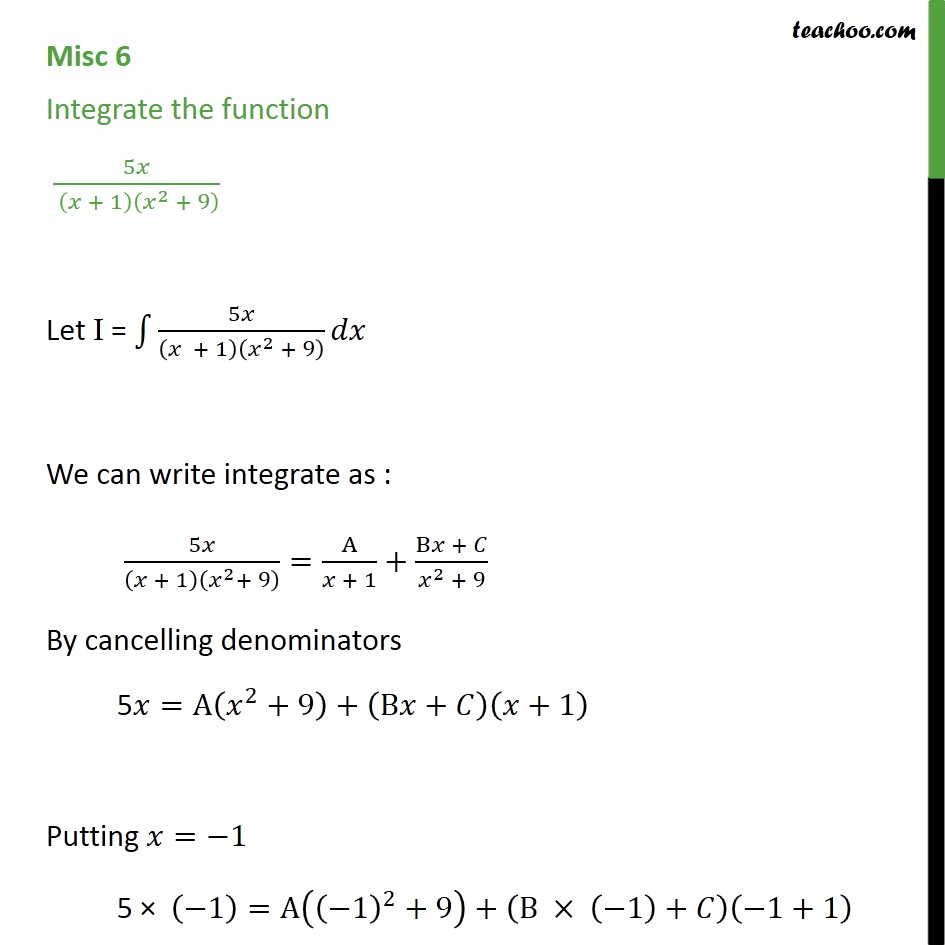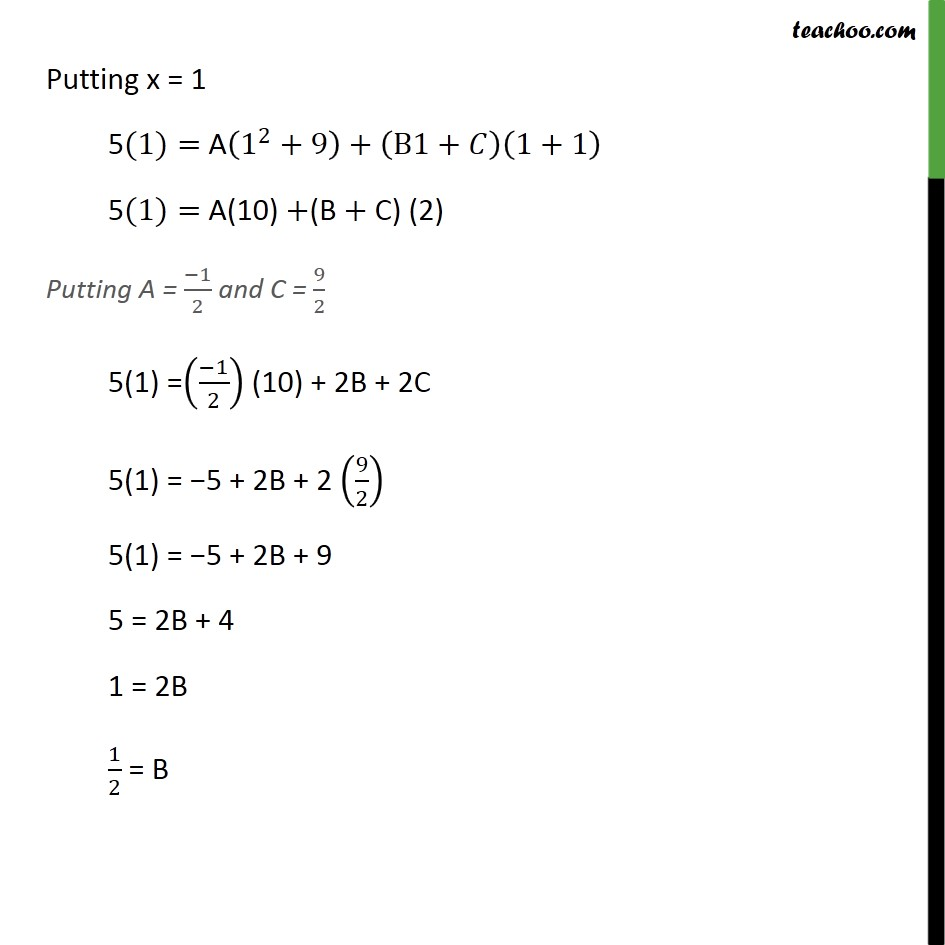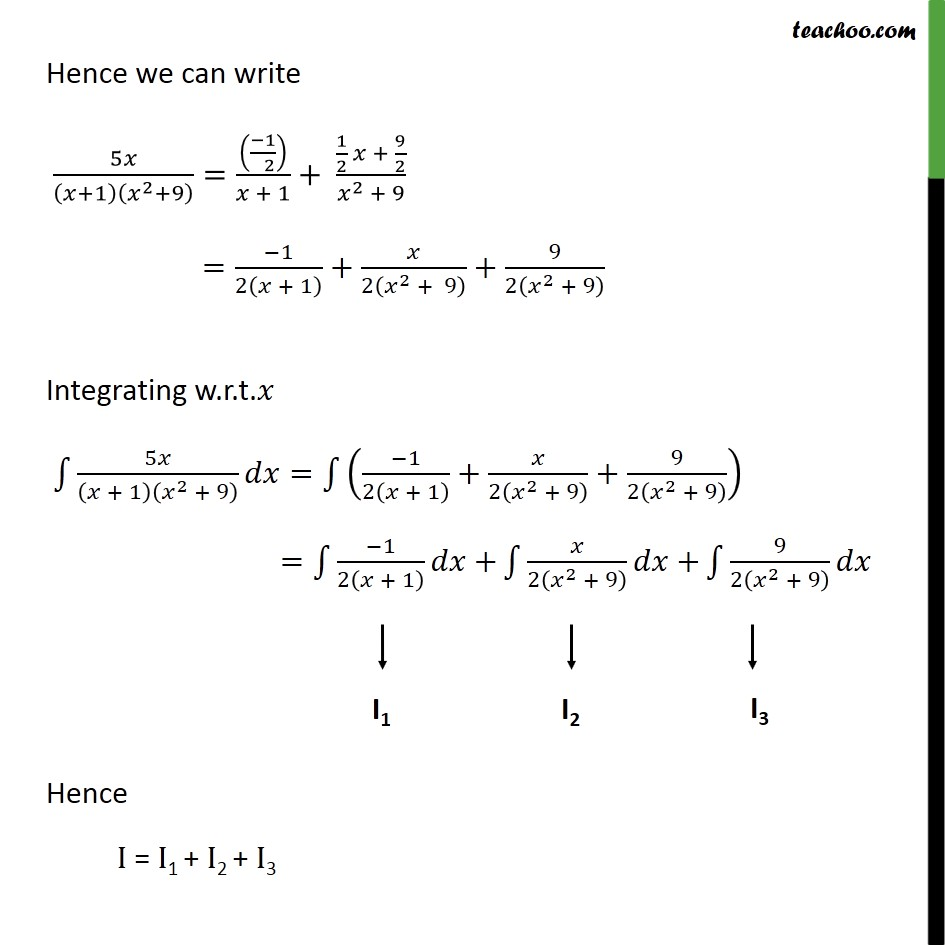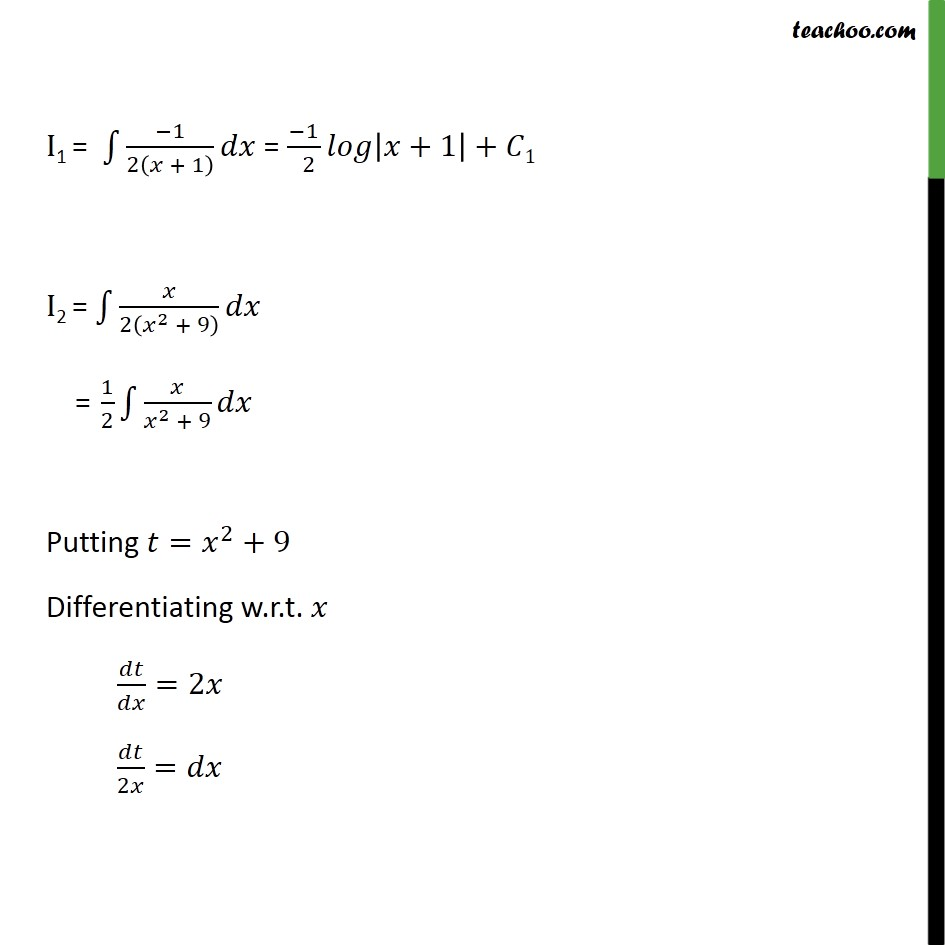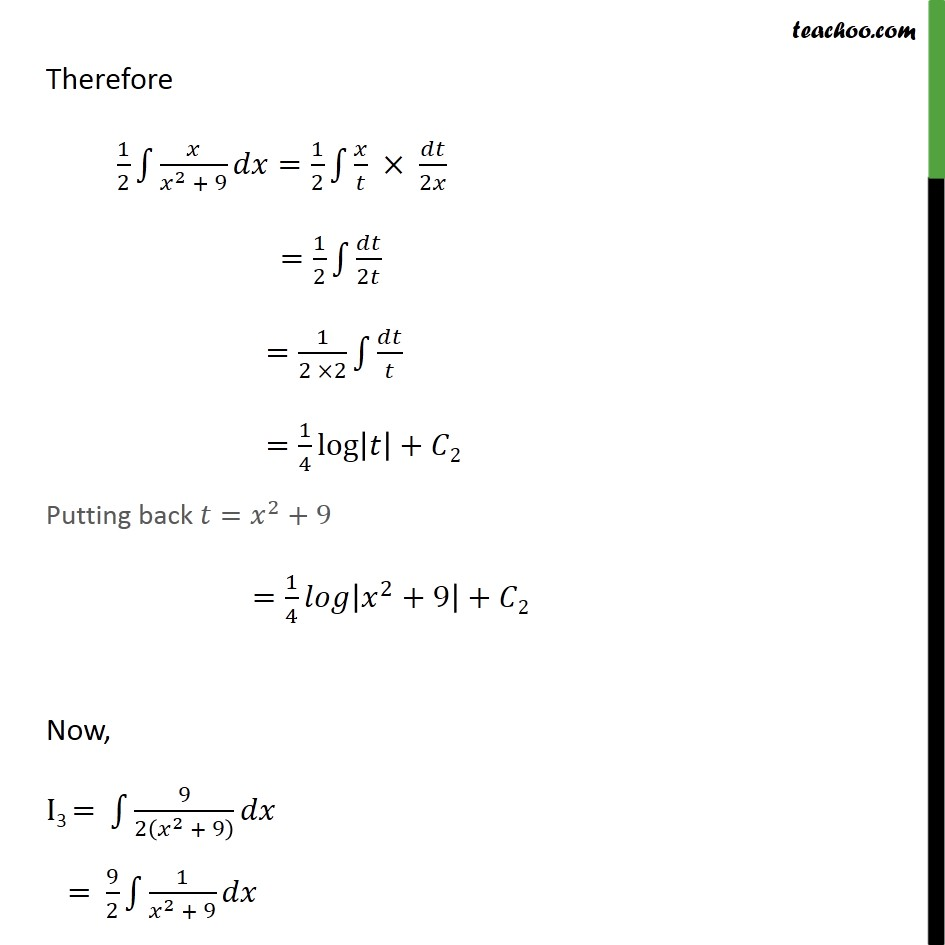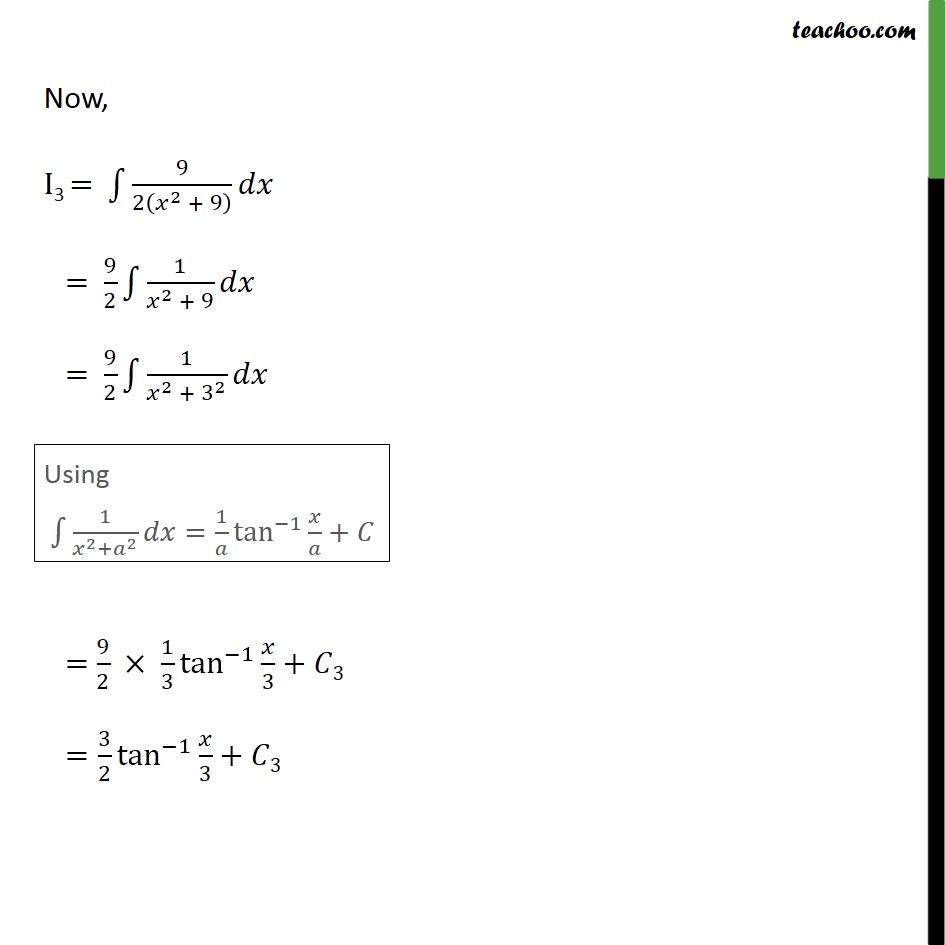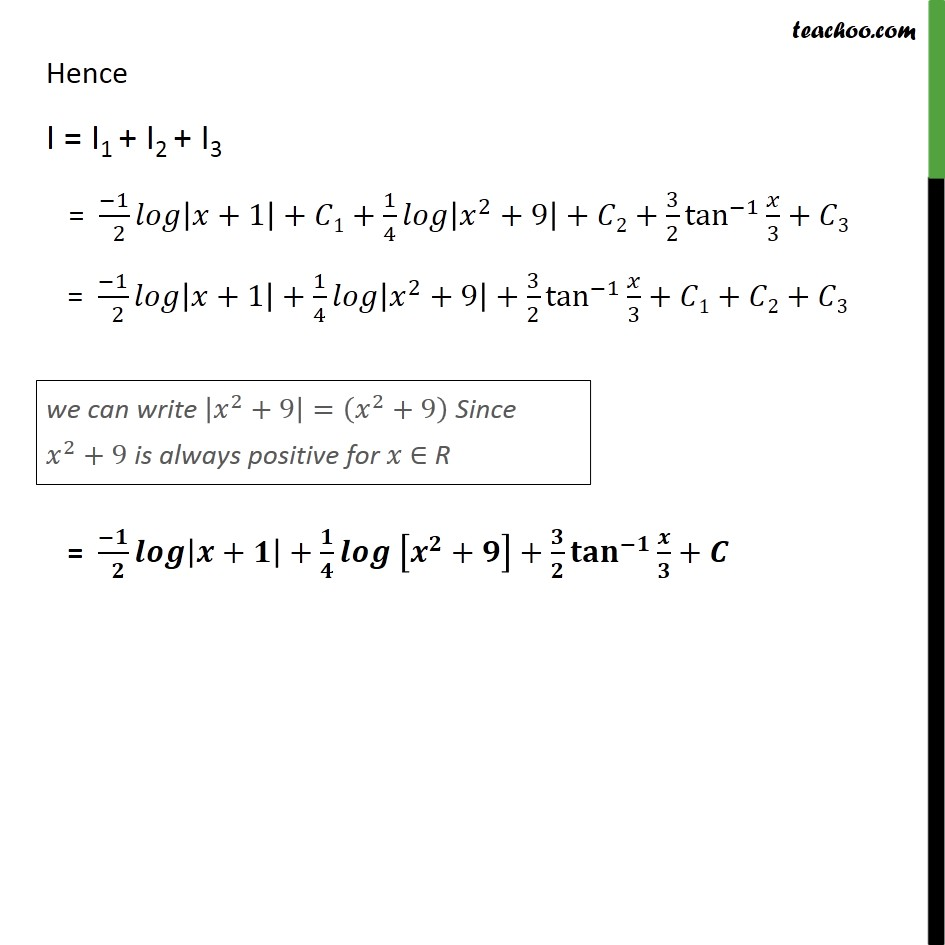Learn in your speed, with individual attention - Teachoo Maths 1-on-1 Class

### Transcript

Misc 6 Integrate the function 5𝑥﷮ 𝑥 + 1﷯ 𝑥﷮2﷯ + 9﷯﷯ Let I = ﷮﷮ 5𝑥﷮ 𝑥 + 1﷯ 𝑥﷮2﷯ + 9﷯﷯𝑑𝑥﷯ We can write integrate as : 5𝑥﷮ 𝑥 + 1﷯ 𝑥﷮2﷯+ 9﷯﷯= A﷮𝑥 + 1﷯+ B𝑥 + 𝐶﷮ 𝑥﷮2﷯ + 9﷯ By cancelling denominators 5𝑥=A 𝑥﷮2﷯+9﷯+ B𝑥+𝐶﷯ 𝑥+1﷯ Putting 𝑥=−1 5 × −1﷯=A −1﷯﷮2﷯+9﷯+ B × −1﷯+𝐶﷯ −1+1﷯ – 5 = A 1+9﷯+ −B+𝐶﷯ 0﷯ – 5 = A × 10 – 5 = 10A A = −5﷮ 10﷯ A = −1﷮ 2﷯ Similarly putting 𝑥=0 5 × 0 = A 0﷮2﷯+9﷯+ B ×0+𝐶﷯ 0+1﷯ 0 = A 9﷯+ 0+𝐶﷯ 1﷯ 0 = 9A + C C = – 9A C = – 9 × −1﷮ 2﷯ C = 9﷮2﷯ Putting x = 1 5(1)= A 1﷮2﷯+9﷯+ B1+𝐶﷯ 1+1﷯ 5(1)= A(10) +(B + C) (2) Putting A = −1﷮2﷯ and C = 9﷮2﷯ 5(1) = −1﷮2﷯﷯ (10) + 2B + 2C 5(1) = −5 + 2B + 2 9﷮2﷯﷯ 5(1) = −5 + 2B + 9 5 = 2B + 4 1 = 2B 1﷮2﷯ = B Hence we can write 5𝑥﷮ 𝑥+1﷯ 𝑥﷮2﷯+9﷯﷯= −1﷮ 2﷯﷯﷮𝑥 + 1﷯+ 1﷮2﷯ 𝑥 + 9﷮2﷯﷮ 𝑥﷮2﷯ + 9﷯ = −1﷮2 𝑥 + 1﷯﷯+ 𝑥﷮2 𝑥﷮2﷯ + 9﷯﷯+ 9﷮2 𝑥﷮2﷯ + 9﷯﷯ Integrating w.r.t.𝑥 ﷮﷮ 5𝑥﷮ 𝑥 + 1﷯ 𝑥﷮2﷯ + 9﷯﷯𝑑𝑥= ﷮﷮ −1﷮2 𝑥 + 1﷯﷯+ 𝑥﷮2 𝑥﷮2﷯ + 9﷯﷯+ 9﷮2 𝑥﷮2﷯ + 9﷯﷯﷯﷯﷯ = ﷮﷮ −1﷮2 𝑥 + 1﷯﷯𝑑𝑥+﷯ ﷮﷮ 𝑥﷮2 𝑥﷮2﷯ + 9﷯﷯𝑑𝑥+﷯ ﷮﷮ 9﷮2 𝑥﷮2﷯ + 9﷯﷯﷯𝑑𝑥 Hence I = I1 + I2 + I3 I1 = ﷮﷮ −1﷮2 𝑥 + 1﷯﷯𝑑𝑥﷯ = −1﷮ 2 ﷯𝑙𝑜𝑔 𝑥+1﷯+𝐶1 I2 = ﷮﷮ 𝑥﷮2 𝑥﷮2﷯ + 9﷯﷯𝑑𝑥﷯ = 1﷮2﷯ ﷮﷮ 𝑥﷮ 𝑥﷮2﷯ + 9﷯𝑑𝑥﷯ Putting 𝑡= 𝑥﷮2﷯+9 Differentiating w.r.t. 𝑥 𝑑𝑡﷮𝑑𝑥﷯=2𝑥 𝑑𝑡﷮2𝑥﷯=𝑑𝑥 Therefore 1﷮2﷯ ﷮﷮ 𝑥﷮ 𝑥﷮2﷯ + 9﷯𝑑𝑥﷯= 1﷮2﷯ ﷮﷮ 𝑥﷮𝑡﷯ × 𝑑𝑡﷮2𝑥﷯﷯ = 1﷮2﷯ ﷮﷮ 𝑑𝑡﷮2𝑡﷯﷯ = 1﷮2 ×2﷯ ﷮﷮ 𝑑𝑡﷮𝑡﷯﷯ = 1﷮4﷯log 𝑡﷯+𝐶2 Putting back 𝑡= 𝑥﷮2﷯+9 = 1﷮4﷯𝑙𝑜𝑔 𝑥﷮2﷯+9﷯+𝐶2 Now, I3 = ﷮﷮ 9﷮2 𝑥﷮2﷯ + 9﷯﷯﷯𝑑𝑥 = 9﷮2﷯ ﷮﷮ 1﷮ 𝑥﷮2﷯ + 9﷯𝑑𝑥﷯ Now, I3 = ﷮﷮ 9﷮2 𝑥﷮2﷯ + 9﷯﷯﷯𝑑𝑥 = 9﷮2﷯ ﷮﷮ 1﷮ 𝑥﷮2﷯ + 9﷯𝑑𝑥﷯ = 9﷮2﷯ ﷮﷮ 1﷮ 𝑥﷮2﷯ + 3﷮2﷯﷯𝑑𝑥﷯ = 9﷮2﷯ × 1﷮3﷯ tan﷮−1﷯﷮ 𝑥﷮3﷯+𝐶3﷯ = 3﷮2﷯ tan﷮−1﷯﷮ 𝑥﷮3﷯+﷯ 𝐶3 Hence I = I1 + I2 + I3 = −1﷮ 2﷯𝑙𝑜𝑔 𝑥+1﷯+𝐶1+ 1﷮4﷯𝑙𝑜𝑔 𝑥﷮2﷯+9﷯+𝐶2+ 3﷮2﷯ tan﷮−1﷯﷮ 𝑥﷮3﷯+𝐶3﷯ = −1﷮ 2﷯𝑙𝑜𝑔 𝑥+1﷯+ 1﷮4﷯𝑙𝑜𝑔 𝑥﷮2﷯+9﷯+ 3﷮2﷯ tan﷮−1﷯﷮ 𝑥﷮3﷯+𝐶1+𝐶2+𝐶3﷯ = −𝟏﷮ 𝟐﷯𝒍𝒐𝒈 𝒙+𝟏﷯+ 𝟏﷮𝟒﷯𝒍𝒐𝒈 𝒙﷮𝟐﷯+𝟗﷯+ 𝟑﷮𝟐﷯ 𝐭𝐚𝐧﷮−𝟏﷯﷮ 𝒙﷮𝟑﷯+𝑪﷯